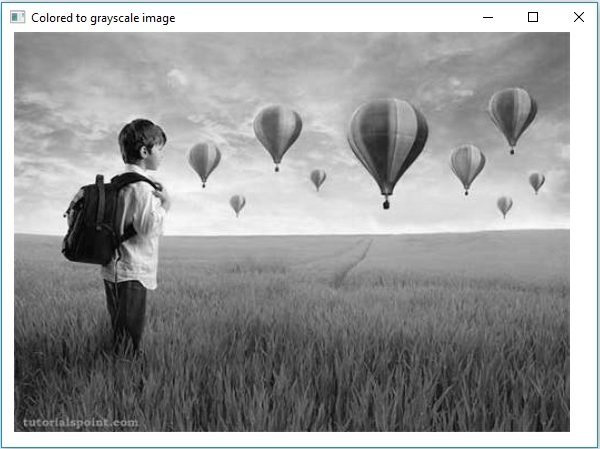# OpenCV - Colored Images to GrayScale

In the earlier chapters, we discussed how to read an input image as different types (binary, grayscale, BGR, etc.). In this chapter, we will learn how to convert one type of image to another.

The class named Imgproc of the package org.opencv.imgproc provides methods to convert an image from one color to another.

## Converting Colored Images to Grayscale

A method named cvtColor() is used to convert colored images to grayscale. Following is the syntax of this method.

```cvtColor(Mat src, Mat dst, int code)
```

This method accepts the following parameters −

• src − A matrix representing the source.

• dst − A matrix representing the destination.

• code − An integer code representing the type of the conversion, for example, RGB to Grayscale.

You can convert colored images to gray scale by passing the code Imgproc.COLOR_RGB2GRAY along with the source and destination matrices as a parameter to the cvtColor() method.

### Example

The following program demonstrates how to read a colored image as a grayscale image and display it using JavaFX window.

```import java.awt.image.BufferedImage;

import org.opencv.core.Core;
import org.opencv.core.Mat;
import org.opencv.imgcodecs.Imgcodecs;
import org.opencv.imgproc.Imgproc;

import javafx.application.Application;
import javafx.embed.swing.SwingFXUtils;
import javafx.scene.Group;
import javafx.scene.Scene;
import javafx.scene.image.ImageView;
import javafx.scene.image.WritableImage;

import javafx.stage.Stage;

public class ColorToGrayscale extends Application {
@Override
public void start(Stage stage) throws Exception {

// Setting the image view
ImageView imageView = new ImageView(writableImage);

// Setting the position of the image
imageView.setX(10);
imageView.setY(10);

// setting the fit height and width of the image view
imageView.setFitHeight(400);
imageView.setFitWidth(600);

// Setting the preserve ratio of the image view
imageView.setPreserveRatio(true);

// Creating a Group object
Group root = new Group(imageView);

// Creating a scene object
Scene scene = new Scene(root, 600, 400);

// Setting title to the Stage
stage.setTitle("Colored to grayscale image");

// Adding scene to the stage
stage.setScene(scene);

// Displaying the contents of the stage
stage.show();
}
public WritableImage loadAndConvert() throws Exception {

String input = "C:/EXAMPLES/OpenCV/sample.jpg";

//Creating the empty destination matrix
Mat dst = new Mat();

//Converting the image to gray sacle and saving it in the dst matrix
Imgproc.cvtColor(src, dst, Imgproc.COLOR_RGB2GRAY);

//Extracting data from the transformed image (dst)
byte[] data1 = new byte[dst.rows() * dst.cols() * (int)(dst.elemSize())];
dst.get(0, 0, data1);

//Creating Buffered image using the data
BufferedImage bufImage = new BufferedImage(dst.cols(),dst.rows(),
BufferedImage.TYPE_BYTE_GRAY);

//Setting the data elements to the image
bufImage.getRaster().setDataElements(0, 0, dst.cols(), dst.rows(), data1);

//Creating a WritableImage
WritableImage writableImage = SwingFXUtils.toFXImage(bufImage, null);
System.out.println("Converted to Grayscale");
return writableImage;
}
public static void main(String args[]) throws Exception {
launch(args);
}
}
```

### Input Image

Assume that following is the input image sample.jpg specified in the above program.### Output Image

On executing the program, you will get the following output.